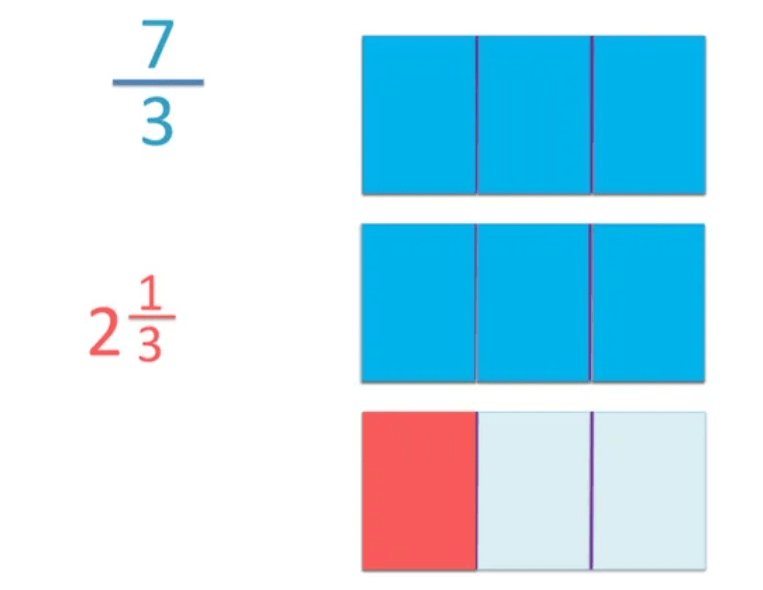# How to Convert Mixed Numbers to Improper Fractions

How to Convert Mixed Numbers to Improper Fractions• A mixed number is a number written as a whole number next to a fraction.
• An improper fraction is a fraction that has a larger numerator on top than its denominator on the bottom.
• 2   2 / 3  is a mixed number and it can be written as an improper fraction as 8 / 3 .
• 2   2 / 3   is the same amount as 8 / 3 .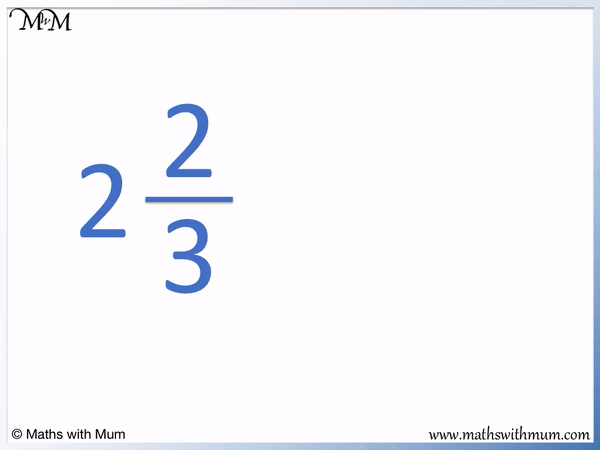• To convert a mixed number to an improper fraction use the following steps.
• Keep the denominator of the improper fraction the same as the denominator of the mixed number.
• The denominator of the improper fraction is 3.
• To find the numerator of the improper fraction, look at the mixed number to multiply the whole number part with the denominator and then add the numerator.
• 2 × 3 = 6 and then 6 + 2 = 8. The numerator is 8.
• The mixed number of 2   2 / 3   is written as an improper fraction as 8 / 3 .

Keep the denominator the same.

To find the numerator, multiply the whole number with the denominator and then add the numerator of the mixed number.• We keep the denominator as 4.
• To find the numerator, we multiply the whole number part with the denominator and then add the numerator of the mixed number.
• 5 × 4 = 20 and then 20 + 1 = 21. The numerator is 21.
• 5   1 / 4   is the same amount as 21 / 4 .# Converting Mixed Numbers to Improper Fractions

## How to Convert Mixed Numbers to Improper Fractions

To convert a mixed number to an improper fraction, follow these steps:

1. Keep the denominator of the improper fraction the same as the denominator of the mixed number.
2. Multiply the whole number part and the denominator part of the mixed number together.
3. Add the numerator of the mixed number to this result to get the numerator of the improper fraction.

A mixed number is a number made up of a whole number and a fraction side by side.

An improper fraction is one fraction that has a larger number as its numerator on top than its denominator on the bottom. By contrast, a proper fraction is a fraction that has a numerator that is less than its denominator.

We can easily convert between a mixed number and an improper fraction.

Here is an example of converting a mixed number to an improper fraction. We have 2   2 / 3  .The first step is to keep the denominator of the mixed number the same as the denominator of the improper fraction.

The denominator of the mixed number is 3 and so, the denominator on the bottom of the improper fraction is also 3.

The second step is to multiply the whole number and denominator of the mixed number together.The whole number part is 2 and the denominator is 3. 2 × 3 = 6.

The third step is to add the numerator of the mixed number to this result.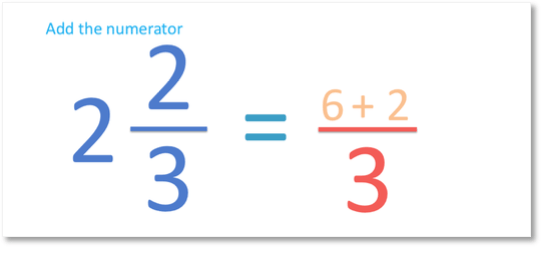The numerator of the mixed number is 2. 6 + 2 = 8 and so, 8 is the numerator on the top of the improper fraction answer.The mixed number of 2   2 / 3  written as an improper fraction is   8 / 3  .

Here is another example of how to convert a mixed number to an improper fraction. We have 3   5 / 7.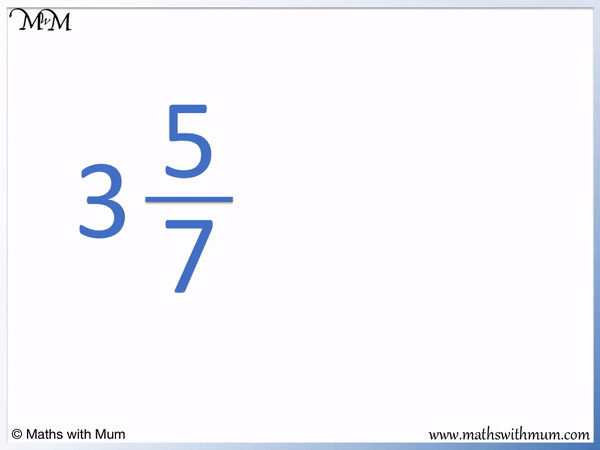The denominator on the bottom of an improper fraction is the same as the denominator of the mixed number. Here, the denominator is 7.

We multiply the whole number with the denominator and then add the numerator to find the numerator of the improper fraction.

3 × 7 = 21 and then 21 + 5 = 26.

The mixed number of 3   5 / 7   can be written as an improper fraction as   26 / 7.

26 is larger than 7 and so, this is called an improper fraction.

## How are Mixed Numbers and Improper Fractions Related?

Mixed numbers and improper fractions are different ways of writing the same amount. An improper fraction is written as one fraction only and it has a larger numerator on top than its denominator on the bottom. A mixed number is written as a whole number alongside a fraction.

We can see in the diagram below that the mixed number of 2   2 / 3   is the same size as the improper fraction   8 / 3  .The whole number part of a mixed fraction tells us how many complete numbers we have.

Here we have 2 complete blocks shown in the diagram.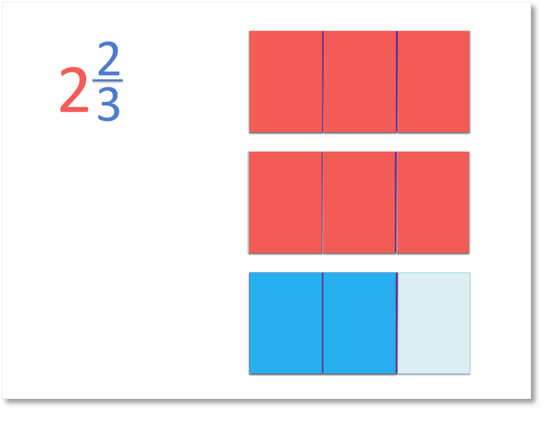The fraction of a mixed fraction tells us how many fractional parts we have.

Here we have two thirds of a block as well.

In a mixed number, the total amount shown is the whole number plus the fractional number.

2   2 / 3   means that we have 2 wholes and two thirds of a whole added together.Instead of counting whole numbers and fractions, we can simply count the amount shown as one fraction. We will count the number of thirds we have in total. We count how many separate pieces there are in the diagram below.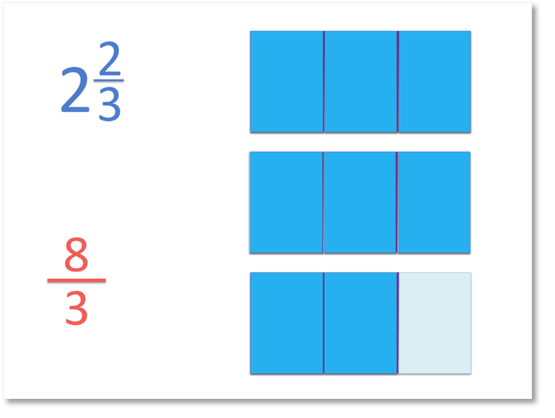In each complete block there are 3 thirds. There are 8 thirds shown in total.

Mixed numbers can always be written as improper fractions.

The relationship between mixed numbers and improper fractions is that the denominator of both is always the same. The whole number and the denominator are multiplied and then added to the numerator of the mixed number to find the numerator of the improper fraction.The reason that the rule for converting mixed numbers to improper fractions works is that each whole number contains the the same number of fractional pieces as the denominator.

For example, each whole number in 2   2 / 3   contains 3 thirds.

Therefore there are 6 thirds in the 2 whole numbers.To find the total number of fractional pieces in each whole number, simply multiply the whole number by the denominator of the fraction.

## Mixed Numbers and Improper Fractions on a Number Line

To write improper fractions on a number line, keep the denominator the same and increase the numerator by 1 at each new increment. To write mixed numbers on a number line, increase the numerator by 1 at each new increment but reset it to zero at each new whole number.

When teaching mixed numbers and improper fractions, it can be helpful to show both types of number either side of a number line. This allows us to compare the two types of number much more easily.

We can convert between mixed numbers and improper fractions by listig them on a number line.

Here is a comparison of mixed numbers and improper fractions on a number line. In this example, we are going up in halves.

We start at 0 and the denominators are 2. We count up in halves. Each increment shown is worth one half.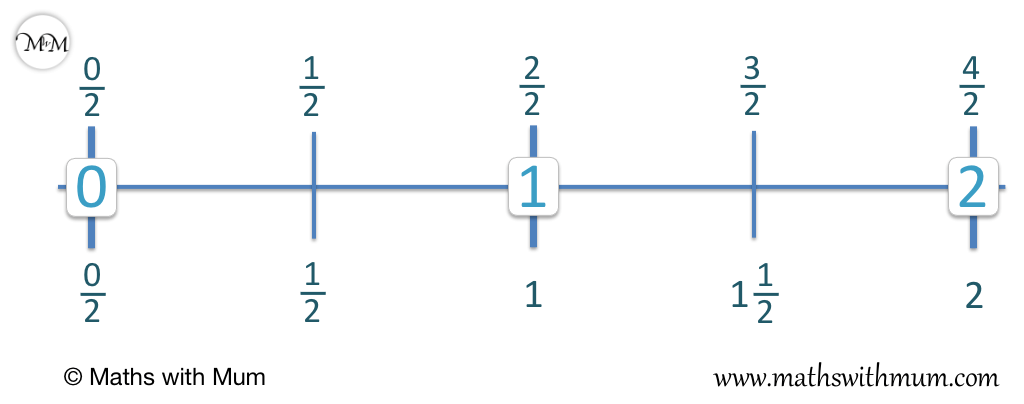We can see that 1 is the same as   2 / 2   and 2 is the same as   4 / 2  .

This is because 4 ÷ 2 = 2. 4 halves are the same as 2 wholes.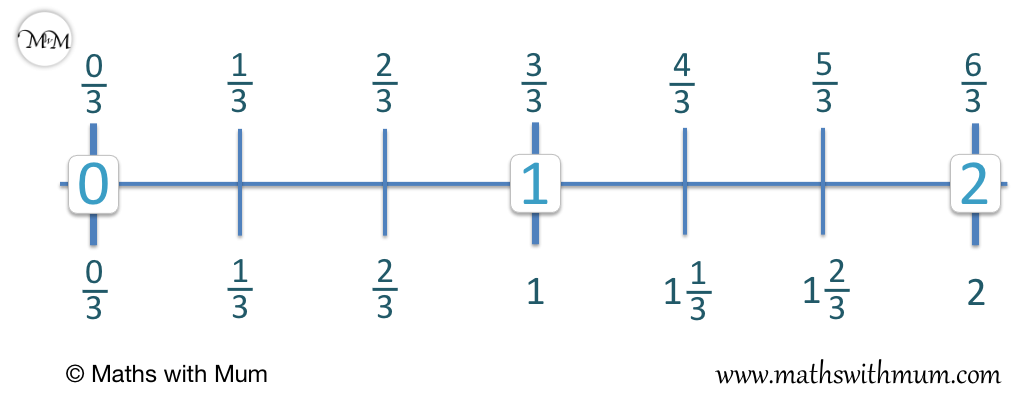We count up in thirds on the number line. We can see that at each whole number, the numerator of the mixed number is reset to 0 and we do not write it.

For example, 1 is the same as   3 / 3   and 2 is the same as   6 / 3  .

Here is an example of comparing mixed numbers and improper fractions on a number line by counting up in quarters.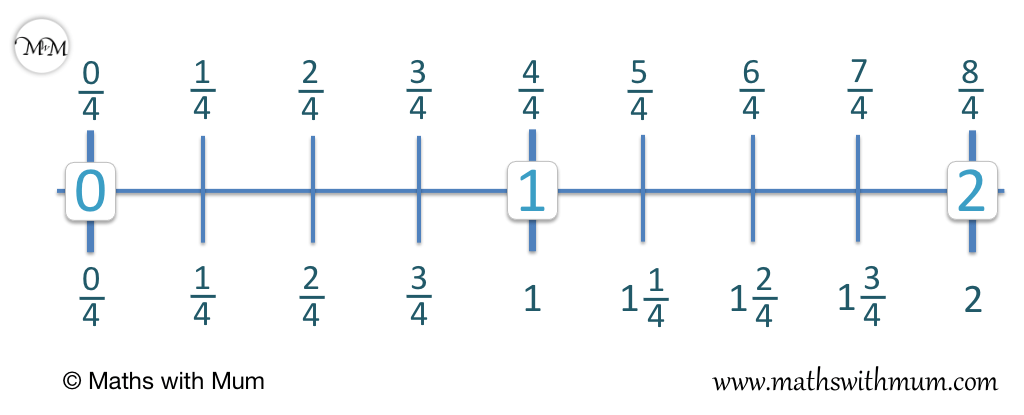We can see how to convert between mixed numbers and improper fractions using a number line.

1 is the same as   4 / 4   and 2 is the same as   8 / 4  .

This is because 8 ÷ 4 = 2. There are 8 quarters in 2 whole numbers.

When teaching how to convert between an improper fraction and a mixed number, diagrams and number lines are helpful tools. Number lines are used to show the comparitive sizes of improper fractions and mixed numbers.

## Why Convert a Mixed Number to an Improper Fraction

It is useful to write a number as a mixed number because the whole number part allows us to better understand the size of the number. It is useful to convert mixed numbers to improper fractions when doing calculations. Improper fractions can be easier to work with in calculations than mixed numbers since they follow the normal fraction rules.

Here is the mixed number 1   3 / 4  .

It is useful to have a number written as a mixed number because the whole number part tells us approximately how large it is.

Since the whole number part is 1, we know that this fraction is larger than 1 but less than 2 simply by looking at it.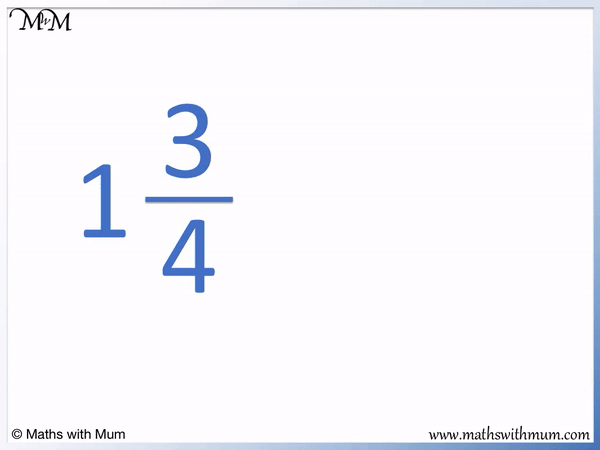We can write this mixed fraction as an improper fraction.

1 × 4 = 4 and 4 + 3 = 7.

1   3 / 4   is the same as   7 / 4  .

We can see that the improper fraction of   7 / 4   does not immediately tell us how large the number is compared to the whole numbers. Without doing any further calculations we cannot see if   7 / 4   is larger than 1, 2, 3 or any other number.

However the fraction   7 / 4   can be easier to work with when doing arithmetic with fractions.

When adding, subtracting, multiplying or dividing fractions we usually convert mixed numbers to improper fractions. They behave just like proper fractions, which are fractions that have a numerator less than the denominator.Now try our lesson on How to Convert Improper Fractions to Mixed Numbers where we learn how to write an improper fraction as a mixed number.